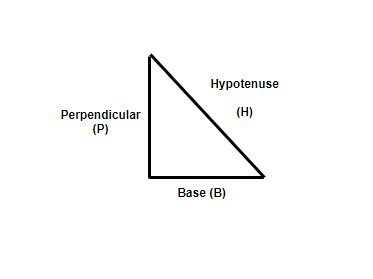GFG App
Open AppBrowser
Continue

# A Pythagorean triplet whose two numbers are 8 and 10, then what is the third number?

A Pythagoras Theorem is defined for the Right-angled triangles. Pythagoras theorem defines relationships among the sides of the right-angled triangle. The theorem is also known as the Pythagorean theorem and is defined as “The square of the hypotenuse is equal to the sum of the squares of the other two sides, perpendicular, and the base.” The perpendicular and base of the right-angled triangle are the two sides that are at 90° to each other and the hypotenuse is known as the third side.

### Pythagoras Formula

The formula is defined with the help of the theorem, the square of the hypotenuse if equal to the sum of the squares of the other two sides of a right angles triangle,(Hypotenuse)2 = Perpendicular2 + Base2

H2 = P2 + BExample: In a right-angled triangle, the values of the two sides that are 90° to each other is given as, m and 3m. Find the value of the third side of the triangle.

Solution:

The sides that are 90° to each other will be perpendicular and base and the third side that is required to be found is the hypotenuse.

Therefore, P=m, B= 3m, H=?

The formula for Pythagoras theorem is,

H2 = P2 +B2

H2 = m2 +(3m)2

H2 = m2 + 9m2

H2 = 10m2

H = √10 m

### A Pythagoras triplet whose two numbers are 8 and 10, then what is the Third number?

The above question is very interesting as the values of two sides are given, but it is not given that if the sides are hypotenuse, perpendicular or base. Let’s say that the triplets are required to be written as (x, 8, 10), x is the unknown triplet.

Since, 10 is the largest number given in the question and hypotenuse is the largest side, lets assume that hypotenuse is 10 units and perpendicular is 8 units.

According to the formula for Pythagoras theorem,

H2 = P2 +B2

102 = 82 + x2

100 = 64 + x2

x2 = 100 – 64

x2 = 36

x = √36

x = 6 units.

Hence, the triplets are (6, 8, 10).

My Personal Notes arrow_drop_up